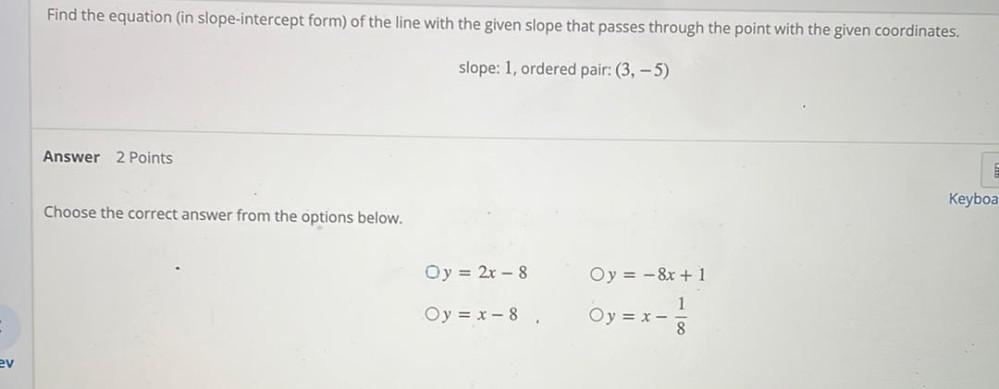Question:

# Find the equation (in slope-intercept form) of the line with the given slope that passes through the point with the given coordinates.slope: 1, ordered pair: (3,-5) Choose the correct answer from theFind the equation (in slope-intercept form) of the line with the given slope that passes through the point with the given coordinates.slope: 1, ordered pair: (3,-5) Choose the correct answer from the options below. (A) y = 2x - 8 (B) y = - 8x + 1 (C) y = x -8 (D) y = x - 1/8